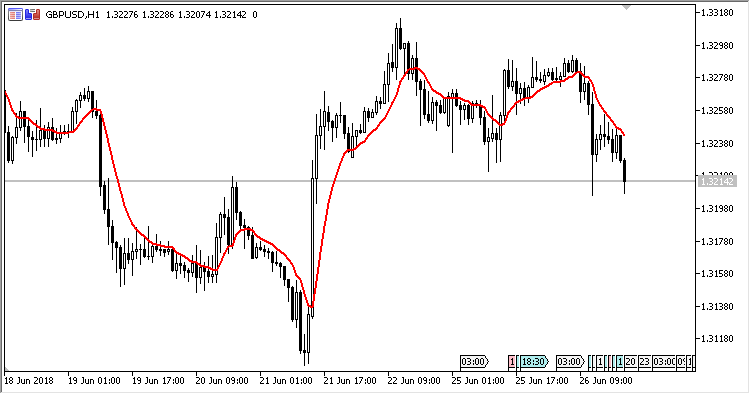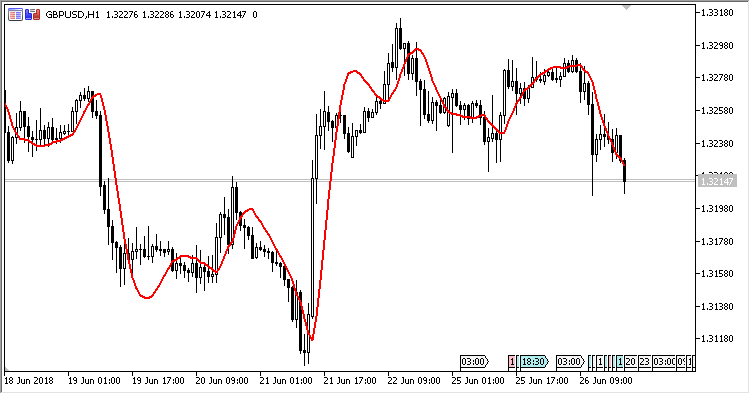# EMA_With_Trend_Adjustment – indicator for MetaTrader 5

• A+
category：MT5 INDICATORS

EMA_With_Trend_Adjustment - a moving average with exponential smoothing and correction by its trend component.

The indicator has four configured parameters:

• Alpha - coefficient to calculate EMA;
• Beta - coefficient to calculate the trend component;
• Use Trend Adjustment - whether to use correction by trend (Yes/No);
• Applied price - price used for calculations.

Calculations:

```EMATA = Alpha * Price + (1-Alpha) * (PrevEMATA + Trend)
Trend = Beta * (EMATA - PrevEMATA) + (1 - Beta) * PrevTrend
```

where:

`Price - SMA(1, Applied price)`Fig 1. Trend component is not used.Fig. 2. Trend component is used.